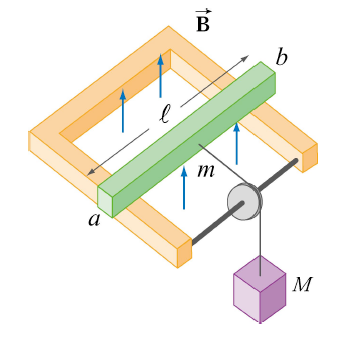# Lenz's Law and GravityA mass $M$ pulls the conducting bar through a vertically oriented magnetic field.

In the above picture, a mass $M$ falls under the influence of gravity, pulling with it a conducting bar of mass $m$, resistance $R$, and length $\ell$ that sits on a U-shaped piece of metal in a vertically oriented magnetic field perpendicular to the plane of the U, pointing in the positive-z direction. In which direction does the induced current flow?

×